Next: Theory of Multivalued Dependencies Up: Normalization Using Multivalued Dependencies Previous: Normalization Using Multivalued Dependencies

## Multivalued Dependencies

1. Functional dependencies rule out certain tuples from appearing in a relation.

If AB, then we cannot have two tuples with the same A value but different B values.

2. Multivalued dependencies do not rule out the existence of certain tuples.

Instead, they require that other tuples of a certain form be present in the relation.

3. Let R be a relation schema, and let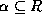and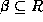.

The multivalued dependency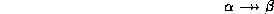holds on R if in any legal relation r(R), for all pairs of tuples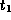and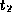in r such that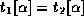, there exist tuples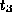and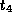in r such that:

```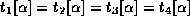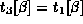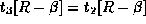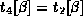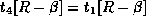```

4. Figure 7.5 (textbook 6.10) shows a tabular representation of this. It looks horrendously complicated, but is really rather simple. A simple example is a table with the schema (name, address, car), as shown in Figure 7.6.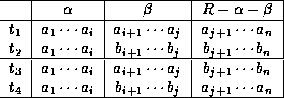Figure 7.5:   Tabular representation of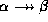.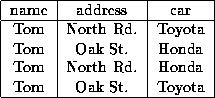Figure 7.6:   (name, address, car) where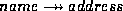and.

• Intuitively,says that the relationship betweenand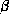is independent of the relationship betweenand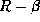.
• If the multivalued dependencyis satisfied by all relations on schema R, then we say it is a trivial multivalued dependency on schema R.
• Thusis trivial if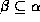or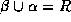.
5. Look at the example relation bc relation in Figure 7.7 (textbook 6.11).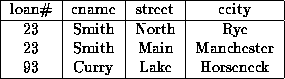Figure 7.7:   Relation bc, an example of redundancy in a BCNF relation.

• We must repeat the loan number once for each address a customer has.
• We must repeat the address once for each loan the customer has.
• This repetition is pointless, as the relationship between a customer and a loan is independent of the relationship between a customer and his or her address.
• If a customer, say ``Smith'', has loan number 23, we want all of Smith's addresses to be associated with that loan.
• Thus the relation of Figure 7.8 (textbook 6.12) is illegal.
• If we look at our definition of multivalued dependency, we see that we want the multivalued dependency

``` cnamestreet ccity

```

to hold on BC-schema.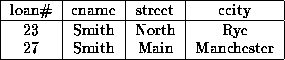Figure 7.8:   An illegal bc relation.

6. Note that if a relation r fails to satisfy a given multivalued dependency, we can construct a relation r' that does satisfy the multivalued dependency by adding tuples to r.Next: Theory of Multivalued Dependencies Up: Normalization Using Multivalued Dependencies Previous: Normalization Using Multivalued Dependencies

Osmar Zaiane
Thu Jun 18 12:56:34 PDT 1998